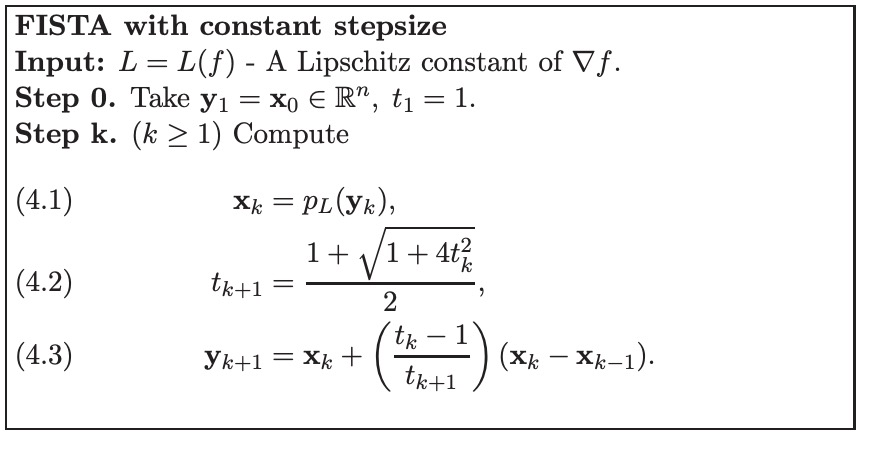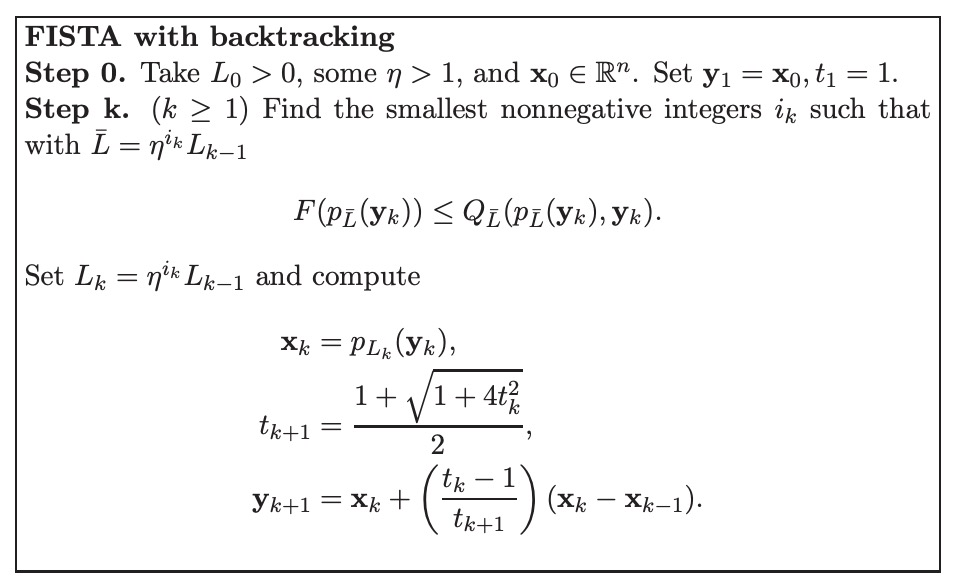Fater Iterative Shrinking Threshold Algorithm

Posted by GwanSiu on October 27, 2018

1. Introduction: Proximal Mapping

The definition of proximal mapping of convex function $h$ is:

$\begin{equation} \textbf{prox}_{h}(x)=\arg\min_{\mu}(h(\mu)+\frac{1}{2}\Arrowvert\mu-x\Arrowvert^{2}) \end{equation}$

2. Faster Iterative Shrinking Threshold Algorithm

The basic idea of the iterative shrinkage algorithm is to build at each iteration a regularization of the linearized differentiable function part in the objective. We consider the following general formualtion:

$\begin{equation} \arg\min_{x}F(x)=f(x)+g(x)\quad x\in\mathcal{R}^{n} \end{equation}$

$g:\mathcal{R}^{n}\rightarrow\mathcal{R}$ is a continuous convex function but is possibly non-smooth. $f:\mathcal{R}^{n}\rightarrow\mathcal{R}$ is a smooth convex function such that Lipschitz continuous gradient $L(f)$:

$\begin{equation} \Arrowvert \nabla f(x)-\nabla f(y)\Arrowvert \leq L(f)\Arrowvert x-y\Arrowvert \end{equation}$

where $\Arrowvert \cdot\Arrowvert$ denotes the euclidean distance and $L(f)$ is the Lipschitz constant of $\nabla f(x)$.

For any $L>0$, we use quadratic upper bound to approximate $F(x)=f(x)+g(x)$ at a given point $y$:

$\begin{equation} F(x)\leq Q_{L}(x,y)=f(y) + \nabla f(x)^{T}(x-y) + \frac{L}{2}\Arrowvert x-y\Arrowvert^{2} + g(x) \end{equation}$

thus, we can use proximal gradient method to compute the minimum value of $Q(x,y)$:

$\begin{equation} \boldsymbol{p}_{L}(y)=\arg\min_{x}g(x)+\frac{L}{2}\Arrowvert x -(y-\frac{1}{L}\nabla(y))\Arrowvert^{2} \end{equation}$

In bothe ISTA and FISTA, update point $x_{k}$ is computed by proximal gradient method:

$\begin{equation} x_{k}=\boldsymbol{p}_{L}(x_{k-1}) \end{equation}$

Faster iterative shrink threshold alorithm is shown as below: